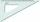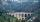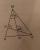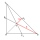# Mirror

How far must Paul place a mirror to see the top of the tower 12 m high? The height of Paul's eyes above the horizontal plane is 160 cm and Paul is from the tower distant 20 m.

Result

x =  2.353 m

#### Solution:Leave us a comment of example and its solution (i.e. if it is still somewhat unclear...):

Showing 0 comments:Be the first to comment!#### To solve this example are needed these knowledge from mathematics:

Do you want to convert length units? See also our right triangle calculator. See also our trigonometric triangle calculator. Try conversion angle units angle degrees, minutes, seconds, radians, grads.

## Next similar examples:

1. Shadow of treeMiro stands under a tree and watching its shadow and shadow of the tree. Miro is 180 cm tall and its shade is 1.5 m long. The shadow of the tree is three times as long as Miro's shadow. How tall is the tree in meters?
2. Double ladderThe double ladder is 8.5m long. It is built so that its lower ends are 3.5 meters apart. How high does the upper end of the ladder reach?
3. RulerHow far from Peter stands 2m hight John? Petr is looking to John over ruler that keeps at arm's distant 60 cm from the eye and on the ruler John measured the height of 15 mm.
4. Spruce heightHow tall was spruce that was cut at an altitude of 8m above the ground and the top landed at a distance of 15m from the heel of the tree?
5. Mountain railwayHeight difference between points A, B of railway line is 38.5 meters, their horizontal distance is 3.5 km. Determine average climb in permille up the track.
6. A boyA boy of height 1.7m is standing 30m away from flag staff on the same level ground . He observes that the angle of deviation of the top of flag staff is 30 degree. Calculate the height of flag staff.
7. Obtuse angleThe line OH is the height of the triangle DOM, line MN is the bisector of angle DMO. obtuse angle between the lines MN and OH is four times larger than the angle DMN. What size is the angle DMO? (see attached image)
8. Double ladderThe double ladder shoulders should be 3 meters long. What height will the upper top of the ladder reach if the lower ends are 1.8 meters apart?
9. ClimbFor horizontal distance 4.9 km road rise by 6.1 m. Calculate the road pitch in ‰ (permille, parts per thousand).
10. Center traverseIt is true that the middle traverse bisects the triangle?
11. AnglesThe outer angle of the triangle ABC at the vertex A is 114°12'. The outer angle at the vertex B is 139°18'. What size is the internal angle at the vertex C?
12. 30-60-90The longer leg of a 30°-60°-90° triangle measures 5. What is the length of the shorter leg?
13. Plan scaleAt what scale is drawn plan of the building, where one side of the building is 45 meters long is on the plan expressed by a straight line 12 mm long.
14. Cable carCable car rises at an angle 45° and connects the upper and lower station with an altitude difference of 744 m. How long is "endless" tow rope?
15. Find the 9Find the missing angle in the triangle and then name triangle. Angles are: 95, 2x+15, x+3
16. Medians 2:1Median to side b (tb) in triangle ABC is 12 cm long. a. What is the distance of the center of gravity T from the vertex B? b, Find the distance between T and the side b.
17. SlopeFind the slope of the line: x=t and y=1+t.